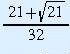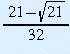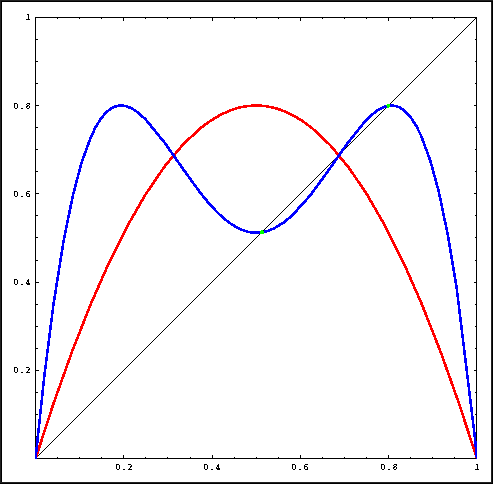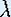Up: Linear and Nonlinear Behavior

# One-Dimensional Dynamical Systems

## Part 4: Linear and Nonlinear Behavior

#### Periodic points

Recall that p is a fixed point of f if f(p) = p. This means that fixed points are the intersection points of the graph of f and the diagonal y = x. This observation makes it easy to find fixed points visually. If we want an exact expression for the fixed point, we need to solve the equation f(p) = p.
• Algebraically verify that +1 and -1 are fixed points of the function f(x) = 1/x.
• Algebraically verify that 0 is a fixed point of the "+/-" function.
• Algebraically find the fixed points of the square root function.

Let fn denote the n-th iterate of f. For example, f2(x) = f(f(x)) is the second iterate of x.

Definition(Periodic Point): We say that p is periodic with least period n, if fk(p) = p for k = n, and this is not true for any smaller value of k.

The orbit of a periodic point p with least period n consists of exactly n different points, since fk(p) = p. Saying "least period" all the time is cumbersome. Therefore, whenever we refer to a period n point, it is assumed to be of least period n.

For example, 1 is a fixed point of the function f(x) = 1/x, but 3 is mapped to 1/3 and 1/3 is mapped back to 3, so 3 is a periodic point of f(x) = 1/x, and its least period is 2. Note that 1/3 is then a periodic point of least period 2 as well.

• What is the period of a point under application of the "+/-" button?

• Check that for f(x) = 3.2 x (1 - x), the points x = 0 and x = 11/16 are fixed points. Check thatandare period 2 points.Points of period 2 for the Logistic map.

The method of finding fixed points graphically also works to find periodic points. For example, to find the points of least period two for a function f, look for the intersection points of the graph of f2 with the line y = x. The only catch is that fixed points are also period two points, but not of least period two. Thus you must first find the fixed points for f and discard them when looking at intersections of the graph of f2 and the line y = x.

For the following questions, read the instructions for the Macintosh or for other machines.

• Draw the graphs of the first and second iterate of the Logistic map for= 3.2. Check graphically the number of points of least period 2. Does this agree with what you found above analytically?

• Graphically determine the number of period two points for functions in the Logistic family at= 2, 3.2, and 3.5. Remember to exclude fixed points when you count intersections.

Just as with fixed points, periodic orbits can be attracting, repelling, or neutral. For a given periodic orbit, if orbits of nearby points converge to the periodic orbit, it is attracting. If orbits of nearby points move away from the periodic orbit, it is repelling. Otherwise it is neutral.

Up: Linear and Nonlinear BehaviorThe Geometry Center Home Page

Written by Hinke Osinga